# KR100956503B1 - Method and device for measuring scattering coefficient of examinee - Google Patents

## Info

Publication number
KR100956503B1
KR100956503B1 KR1020087004315A KR20087004315A KR100956503B1 KR 100956503 B1 KR100956503 B1 KR 100956503B1 KR 1020087004315 A KR1020087004315 A KR 1020087004315A KR 20087004315 A KR20087004315 A KR 20087004315A KR 100956503 B1 KR100956503 B1 KR 100956503B1
Authority
KR
South Korea
Prior art keywords
vector
subject
scalar
measuring
value
Prior art date
Application number
KR1020087004315A
Other languages
Korean (ko)
Other versions
KR20080032223A (en
Inventor
타이치 모리
가쿠 카미타니
Original Assignee
가부시키가이샤 무라타 세이사쿠쇼
Priority date (The priority date is an assumption and is not a legal conclusion. Google has not performed a legal analysis and makes no representation as to the accuracy of the date listed.)
Filing date
Publication date
Priority to JPJP-P-2005-00253158 priority Critical
Priority to JP2005253158 priority
Application filed by 가부시키가이샤 무라타 세이사쿠쇼 filed Critical 가부시키가이샤 무라타 세이사쿠쇼
Publication of KR20080032223A publication Critical patent/KR20080032223A/en
Application granted granted Critical
Publication of KR100956503B1 publication Critical patent/KR100956503B1/en

## Images

•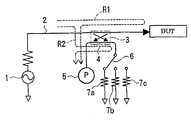•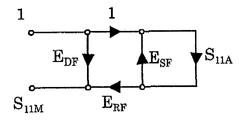•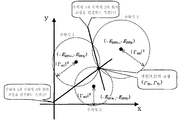•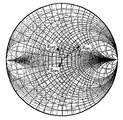•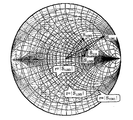•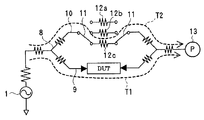•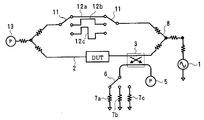•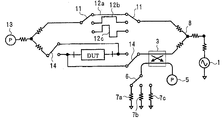•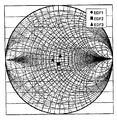•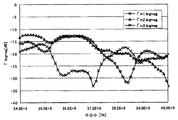•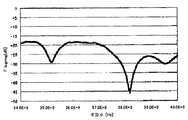•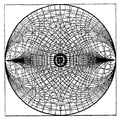•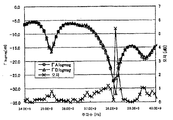•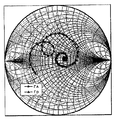••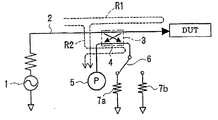••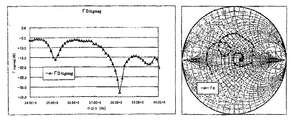•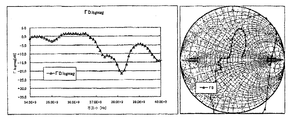•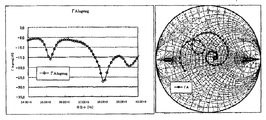## Classifications

• GPHYSICS
• G01MEASURING; TESTING
• G01RMEASURING ELECTRIC VARIABLES; MEASURING MAGNETIC VARIABLES
• G01R27/00Arrangements for measuring resistance, reactance, impedance, or electric characteristics derived therefrom
• G01R27/28Measuring attenuation, gain, phase shift or derived characteristics of electric four pole networks, i.e. two-port networks using network analysers Measuring transient response
• GPHYSICS
• G01MEASURING; TESTING
• G01RMEASURING ELECTRIC VARIABLES; MEASURING MAGNETIC VARIABLES
• G01R27/00Arrangements for measuring resistance, reactance, impedance, or electric characteristics derived therefrom
• G01R27/02Measuring real or complex resistance, reactance, impedance, or other two-pole characteristics derived therefrom, e.g. time constant
• G01R27/04Measuring real or complex resistance, reactance, impedance, or other two-pole characteristics derived therefrom, e.g. time constant in circuits having distributed constants, e.g. having very long conductors or involving high frequencies

## Abstract

The present invention provides a measuring method and a measuring apparatus which can perform a vector measurement of a scattering coefficient of a subject substantially by a scalar measuring device, which can reduce the size of the measuring device and reduce the cost.
The relationship between the signal source 1 for applying a signal to the subject, the scalar measuring instruments 5 and 13 for measuring the reflected or transmitted wave of the subject as a scalar value, and the reflected or transmitted wave of the subject in advance A measuring system having an overlapping signal system each of which superimposes three different valued vector signals is prepared. Three vector signals are superposed on the reflected or transmitted wave of the subject, and each superimposed signal is measured as a scalar value by the scalar measuring instruments 5 and 13, respectively. The measured scalar values are converted into one vector value using the valued relation values of the three vector signals to obtain scattering coefficients of the subject.
Subject, scattering coefficient, reflected wave, transmitted wave, scalar value, vector value, measuring method, measuring device

## Description

Measurement method and measuring apparatus of scattering coefficient of a subject {METHOD AND DEVICE FOR MEASURING SCATTERING COEFFICIENT OF EXAMINEE}

BACKGROUND OF THE INVENTION 1. Field of the Invention The present invention relates to a measuring method and measuring apparatus for vector measuring scattering coefficients of a subject (DUT) such as an electronic device.

As the operating frequency of high frequency electronic circuits becomes higher and higher, electronic devices used in the circuits must also measure accurate electrical characteristics in the high frequency region. Among the high frequency measurement items, there are important reflection coefficients and transmission coefficients. The reflection coefficient is the ratio of the reflected wave reflected from the DUT to the incident wave applied to the DUT. In addition, a transfer coefficient is the ratio of the transmitted wave which permeate | transmitted the DUT with respect to the incident wave applied to a DUT. These are all vector values (or complex numbers). However, in actual use, only their amplitude information (scalar value) is often important.

When the reflected or transmitted waves of the DUT are observed by a measurement system, these usually include a lot of errors of the measurement system. The true characteristics of the DUT are obtained by removing the error of the measurement system from the observation quantity, which requires observing the reflected or transmitted waves as vector values (vector measurement). When only these amplitude information (scalar values) are observed, it is impossible to remove the error of a measurement system correctly. That is, in actual use, although only the amplitudes of the reflection coefficients and the transfer coefficients are important, vector values are required to accurately measure them.

Vector network analyzers (VNAs) are commonly used for vector measurements with high frequency characteristics above millimeter waves. The VNA applies a measurement signal to the DUT to obtain a scattering coefficient (reflection coefficient, transfer coefficient, etc.) matrix of the DUT, and measures the amplitude ratio and the phase difference for each of the measured signals of the reflected and transmitted waves. In other words, the VNA is a measuring device formed by combining a vector detector with a signal source. The conventional VNA is a configuration of a vector detector, and an important part employs a heterodyne detection method using a phase locked loop (PLL) circuit composed of a local oscillator and a mixer.

However, in the configuration of the VNA, as the measurement frequency increases, the price of the remarkably increases due to the increase in the number of stages of the local oscillator and the mixer of the PLL circuit. In addition, as the frequency increases, a loss in frequency conversion and a decrease in the purity of the measured signal also occur, which makes it difficult to perform high precision measurement.

In Non-Patent Document 1, a method of constructing a measurement system that performs four power measurements on incident and reflected waves to perform power measurement and deriving a phase difference from the four power measurement values using system parameters of the measurement system has been proposed. . In this method, a phase difference in which high accuracy measurement becomes difficult as the frequency increases is obtained based on the measurement of a scalar value called power value which is a basic measurement amount in electromagnetic measurement and measurement accuracy is almost independent of frequency. This can eliminate the above-mentioned drawbacks in VNA. However, when performing the reflection measurement, it is necessary to measure four electric powers with four electric power measuring devices, so that the scale of the whole measuring device becomes large, and there is a problem that it costs.

Although the basic measurement principle of patent document 1 is the same as that of the nonpatent document 1, the measurement accuracy was improved by increasing the power measurement value from four to five, and making it possible to compare the amplitude ratio of an incident wave and a reflected wave. In this method, when performing the reflection measurement, five powers are measured by five power meters, so that the entire scale of the measuring instrument becomes larger and more expensive.

[Non-Patent Document 1] G.F.Engen, "The six-port reflectometer: An alternative network analyzer", IEEE Trans. Microw. Theory Tech., Vol. MTT-25, no. 12, pp lO75-1080, Dec. 1977.

[Patent Document 1] Japanese Patent No. 3540797

Therefore, an object of a preferred embodiment of the present invention is to provide a measuring method and a measuring apparatus which can perform a vector measurement of scattering coefficients of a subject by a scalar measuring device, and can reduce the cost while the measuring device is small in size. Is in.

According to a first preferred embodiment of the present invention, in a measurement method of performing a vector measurement of a scattering coefficient of a subject, a signal source for applying a signal to the subject and a reflected or transmitted wave of the subject are measured as a scalar value. A first step of preparing a measuring system having a scalar measuring device and an overlapping signal system each superimposed on at least three different vector signals with respect to the reflected or transmitted waves of the subject; and the at least three vector signals A second step of measuring a relation value as a vector value and superimposing the at least three vector signals on the reflected or transmitted wave of the subject, and measuring each superimposed signal as a scalar value by the scalar measuring instrument, respectively. The at least three scalar values measured in the said 3rd step are made into at least 1 using the 3rd step and the relationship value obtained by the said 2nd step. By conversion of the vector value, a measuring method, it characterized in that a step of claim 4 to obtain a scattering coefficient of the subject.

According to a second preferred embodiment of the present invention, in a measuring apparatus for performing vector measurement of a scattering coefficient of a subject, a signal source for applying a signal to the subject and a reflected or transmitted wave of the subject are mutually determined. A superimposed signal system in which at least three different vector signals each having a relation value valued as a vector value, respectively, and a superimposed signal in which the at least three vector signals are superimposed with respect to the reflected or transmitted waves of the subject are respectively measured as scalar values. A converting means for converting at least three scalar values measured by the scalar measuring device into at least one vector value by using a scalar measuring device and the relational values of the at least three vector signals to obtain scattering coefficients of the subject. It is a measuring apparatus characterized by having.

The basic idea of the present invention is to perform at least three scalar measurements with different states of the measurement system when measuring the reflected and / or transmitted waves of the DUT, and mathematically obtaining a vector value, that is, a scattering coefficient from at least three scalar values obtained. . To carry out the measurement, a measurement system having a switchable signal path is prepared so that at least three different vector signals can be superimposed on the reflected and / or transmitted waves. The relation value of each vector signal is previously vectorized. Here, as the vector signals that can be superimposed, there are directional errors and leakage errors, for example, but it is not necessary to value the phase or the absolute position of each vector signal, and it is necessary to know the mutual relationship. Next, by using the measuring system, the reflected wave and / or transmitted wave when the three vector signals are switched with respect to the DUT are measured by scalar, whereby the superimposed signal of the reflected wave and / or transmitted wave and the vector signal by the DUT as a scalar value. It can be measured. The reflection coefficient (vector value) or transfer coefficient (vector value) of the DUT can be calculated by calculating the relationship between the superimposed scalar measurement value and the three vector signals.

According to the present invention, one scattering coefficient is derived from a measured relational value (vector value) of three vector signals and a measured value measured as a scalar value of an overlapping signal in which three vector signals are superimposed on a reflected or transmitted wave of a subject. It is to find (vector value). This scattering coefficient is obtained as the intersection of three circles having a center corresponding to the relational value valued in the complex plane and a radius corresponding to the measured scalar value. There is a possibility that a plurality of intersections occur without crossing. In that case, the final scattering coefficient (vector value) can be obtained, for example, by finding the center of three intersections located in a predetermined circle.

In the present invention, the relationship between three vector signals (e.g., directional error and leakage error) needs to be valued in a vector manner. Therefore, a vector measuring device (e.g., VNA) may be used. It is also possible to derive using only a scalar meter by following a unique calibration procedure as described above. The value of the relation value of three vector signals needs to be performed only once in the manufacture of this measuring apparatus, and the relation value which is valued may be used for the subsequent measurement of a DUT. If the DUT changes, you do not need to revalue it.

The present invention is a basic and inexpensive measurement of scalar value (power measurement by power meter or power sensor, voltage measurement by voltmeter, current measurement by ammeter) which is almost independent of frequency. It is evident that vector measurements (scattering coefficients) of reflected and / or transmitted waves are obtained. Here, a scalar measuring device which performs a scalar measurement is a measuring device which measures only amplitude information (a magnitude of amplitude) of power, a voltage, or an electric current. In the high frequency device with many kinds / small quantity production, if it is an inexpensive measuring device by this invention, since it becomes possible to provide this for every device, the burden of processes, such as order change, can be made small. The above feature brings about a very large effect on securing measurement reproducibility in the high frequency measurement of millimeter wave or more, which requires delicate adjustment of the measurement system. In addition, the present invention enables vector error correction while suppressing an increase in the price of the measurement system as long as power measurement, which is basically a thermal measurement, is possible. Since the method of the present invention is not a multiplication phase detection method by a mixer or a sampler, but an addition type phase detection method using a change in measured value at the time of load variation, it is essentially limited to the input bandwidth. There is no. Therefore, there is a possibility that it can be extended to the frequency range of light.

By superimposing at least three vector signals with respect to the reflected or transmitted waves of the subject, by providing at least three directional errors or leakage errors, and directional error switching means or leakage error switching means for selectively switching these errors, Three states can be easily obtained. And in order to obtain the measured value of three states, it is not necessary to prepare a scalar measuring device for each state, and it is enough as one. Therefore, unlike the system which requires many power measuring devices like Nonpatent literature 1 and patent document 1, the magnitude | size of the whole measuring apparatus can be made small and cost can also be reduced.

The reflection coefficient measuring system provides a coupler (directional coupler) in the middle of the measurement signal path connecting the signal source and the DUT, and in at least three states in one port of the other signal path coupled by the coupler with the measurement signal path. By attaching a switchable directional error and connecting the scalar measuring device for measuring a reflected wave to the other port, it can be easily configured. On the other hand, the transfer coefficient measuring system can be easily configured by connecting a signal path having a DUT and a signal path having a leakage error switchable to at least three states in parallel between a signal source and a scalar measuring device for measuring transmission waves. In addition, a combination of the reflection coefficient measuring system and the transmission coefficient measuring system may be configured to configure a one-pass two-port measuring system, or a full two-port measuring system, a full three-port measuring system, or the like may be configured by switching a signal source with a switch. In that case, the scattering coefficients of both the reflection coefficient and the transfer coefficient can be measured simultaneously by switching the directional error and the leakage error by the switching means while the DUT is connected to the measurement system.

In the above description, the method of superimposing at least three vector signals with respect to the reflected or transmitted waves of the subject is described, but it is also possible to use a method of superimposing two vector signals. In this case, since there are only two vector signals as overlapping signals, two vector values are basically derived, so that scattering coefficients (vector values) of the subject can not be determined as one. However, if the schematic characteristics of the scattering coefficient of the subject are known in advance, only one of them can be selected from the two transformed vector values, so that the scattering coefficient of the subject can be obtained from the values. In addition, when the two derived vector values do not fall within the range of the reference characteristic of the subject sample (for example, within a good range), it can be judged as a defective product at the time of mass production screening. Since this method ends with two scalar measurements compared with the above example which requires three scalar measurements, the time required for deriving scattering coefficients is shortened.

Effect of the Invention

As described above, in the first embodiment of the present invention, when measuring the reflected wave and / or transmitted wave of the DUT, at least three scalar value measurements in which the state of the measurement system is different are performed, and from at least three scalar value measured values obtained. It is to calculate scattering coefficient mathematically. In other words, the scattering coefficients of the reflected and / or transmitted waves in the high frequency region can be obtained by the scalar measurement, which is a basic and inexpensive measurement that is almost independent of the frequency. There is no problem that the price is significantly increased, and as the frequency is increased, there is no cause of loss in frequency conversion and deterioration of the measured signal purity. Moreover, since the number of scalar measuring instruments can be reduced compared with the vector measuring method using the conventional power measuring instrument, the magnitude | size of the whole measuring apparatus can be made small and cost can also be reduced.

In the second embodiment of the present invention, two vector signals are superimposed on the reflected and / or transmitted waves of the DUT, each superimposed signal is measured as a scalar value by a scalar measuring instrument, respectively, and these two scalar values are determined by two. By converting into two vector values using the relation value of the vector signal, the scattering coefficient of the subject can be obtained from one of these vector values, so that the number of scalar measurements can be reduced, and the measurement time can be shortened.

1 is a circuit diagram for measuring a reflection coefficient according to a first embodiment of the present invention.

2 is an error model diagram of the return loss measurement.

FIG. 3 is a diagram for explaining a method for deriving a vector measured value from three scalar measured values and directional error.

4 is a Smith chart showing three directional errors that are priced.

5 is a Smith chart in which the superimposed signal of the reflected wave of the DUT and the reflected wave of the directional error is measured.

6 is a circuit diagram for measuring a transfer coefficient according to a second embodiment of the present invention.

Fig. 7 is a circuit diagram showing an example of a one-pass two-port measurement system combining a reflection measurement system and a transmission measurement system according to a third embodiment of the present invention.

Fig. 8 is a circuit diagram showing an example of a full two-port measurement system combining a reflection measurement system and a transmission measurement system according to a fourth embodiment of the present invention.

9 is a Smith chart showing a relationship between three directional errors measured by a vector measuring instrument.

It is a figure which shows the DUT scalar measured value in three cases where a directional error differs.

11 is a diagram showing a vector measurement value (amplitude) of the DUT calculated using the method of the present invention.

12 is a Smith chart showing the vector measurements of the DUT calculated using the method of the present invention.

Figure 13 is a comparison of the amplitude of the measured value (Γ A) of the DUT using a calibrated VNA and a measure (Γ D) of the DUT according to the present invention how the graph.

14 is a Smith chart comparing the measured value (Γ D ) of the DUT according to the present invention with the measured value (Γ A ) of the DUT using a calibrated VNA.

FIG. 15 is a diagram illustrating a method of obtaining a relationship value of three directional errors by a scalar measuring device in a complex plane in a fifth embodiment of the present invention. FIG.

16 is a circuit diagram for measuring a reflection coefficient according to the sixth embodiment of the present invention.

FIG. 17 shows a Smith chart in which the two vector measurement values of the derived test object and known characteristics are plotted in the sixth embodiment of the present invention.

It is a figure which shows the amplitude and Smith chart of the DUT calculated | required from the measured value of one of 6th Embodiment of this invention.

It is a figure which shows the amplitude and Smith chart of the DUT calculated | required from the measured value of the other of 6th Embodiment of this invention.

20 is a diagram showing an amplitude and Smith chart of a known DUT for selecting a true value of the sixth embodiment of the present invention.

EMBODIMENT OF THE INVENTION Below, preferred embodiment of this invention is described, referring drawings.

<Preferred Embodiment 1>

1 shows an apparatus for measuring reflection coefficients of a DUT according to a first preferred embodiment of the present invention. The measurement signal generated by the signal source 1 consisting of the oscillator is applied to the DUT through the measurement signal path 2. In the middle of the measurement signal path 2, the coupler 3 which isolate | separates a part of the signal which flows through this signal path 2 is provided. A power measuring device 5 such as a power meter for measuring the reflected wave as a scalar value is connected to a port on the signal source side of the error signal path 4 coupled with the measurement signal path 2 by the coupler 3. Three types of directional errors 7a to 7c are connected to the port on the DUT side of the signal path 4 via the directional selector switch 6. Directional errors 7a to 7c are phases of reflection different from each other, and obtain a relation value (relative vector value) of the directional error in advance.

2 shows an error model of the return loss measurement. Here, S 11A is the return loss of the DUT, S llM is the measurement of the reflection loss, E DF is the directional error, E RF is the reflection frequency response error, and E SF is the source match error. From the error model of FIG. 2, the relationship between the reflection loss S 11A and the measured value S 11M of the subject is as follows.

Here, the return loss (S 11A ) to be measured for many DUTs is about -15 dB to -20 dB, and E SF can be reduced to -20 dB to -25 dB by incorporation of a probe. Can be. Therefore, E SF S 11A is -35 kV to -45 kV, which is a very small value compared to the measured signal level O k, so it can be approximated by the following equation.

The error due to E RF in Equation (2) can be corrected by obtaining a correction coefficient from a scalar measurement value of a pre-valued DUT. However, a normal scalar measurement cannot be used to correct the directional error (E DF ). . Therefore, in the present invention, the following steps are performed to correct the directional error E DF while being a scalar measurement. In addition, if Γ T from which E DF is actually removed from S 11M can be obtained, Γ T is a vector measurement value, so that the error of E RF and E SF can be eliminated by performing a normal error elimination procedure. In other words, the above assumption is not necessary, and the present invention can be applied even if E SF S 11A cannot be ignored.

Next, the reflection coefficient measuring method of the DUT will be described.

Value of Directional Error

In order to obtain the relation values E DF1 , E DF2 , and E DF3 of the three directional errors 7a to 7c, for example, the measured VNA may be measured, or the measurement may not be performed with the VNA. The vector relation values of three directional errors can be obtained by the calibration procedure. For example, when a relationship value of three directional errors is obtained using a two-port VNA, an antireflection terminal is prepared together with the VNA. Then, the port 1 of the VNA is connected to the signal source side of FIG. 1, the non-reflective termination is connected to the DUT side, and the port 2 of the VNA is connected to the power meter side. In this state, the three measured values S 21 of the transfer coefficient when the directional selector switch 6 is switched to three positions become the relation values of the three directional errors. The three directional errors valued in this way are usually unchanged once the measuring device is manufactured, so that the values can be continuously used after the measuring device is manufactured, so that the measuring device can be used continuously. In the device mass production process, VNA is unnecessary.

Scalar measurement

After completion of the measurement of the directional errors 7a to 7c, reflection measurement is performed by connecting the DUT to the measuring device shown in FIG. That is, the electric power value (scalar value) of the reflected wave in three states at the time of switching the directional changeover switch 6 is measured by the electric power meter 5. The measured measurement value is the amplitude of the superimposition signal of the reflected wave Rl of the DUT shown in FIG. 1 and the reflected wave R2 of the directional errors 7a to 7c.

Conversion from scalar value to vector value

Three vector values (E DF1 , E DF2 , E DF3 ) of pre-valued directional error and three power values (│Γ m1 │, │Γ m2 │, │Γ m3 │) measured by the scalar measurement are obtained. By using this method, it is converted into one vector value, that is, the reflection coefficient Γ T of the DUT. The derivation of the conversion equation will be described below.

When the real part and imaginary part of a vector value are represented by the subscripts x and y, (E DF1 , E DF2 , E DF3 ) and Γ T are as follows.

E DF1 = (E DF1x , E DF1y )

E DF2 = (E DF2x , E DF2y )

E DF3 = (E DF3x , E DF3y )

Γ T = (Γ Tx , Γ Ty )

Three directional errors (E DF1 , E DF2 , E DF3 ), reflection coefficient scalar measurements (│Γ m1 │, │Γ m2 │, │Γ m3 │) of the three DUTs, and vector measurement without directional errors, respectively since the value of relation (Γ T) is a vector measured value (Γ T) a value that is taking (x, y) to when the amplitude reflection coefficient of a point on vector measurement plus the directional error scalar measure, mathematics It is expressed by Equations 3 to 5.

In the following, based on the assumption that the vector measured value (T Γ) is present, which satisfies the following expression 3 to 5 at the same time in order to derive a vector measurement value (Γ T) satisfying the three equations. When these three simultaneous equations are solved by a conventional method, four solutions are obtained, and the two corresponding solutions become vector measurement values Γ T. However, in this method, since the calculation amount for obtaining four solutions is huge, it is easy to increase the error by rounding up the calculator. Therefore, the vector measurement value (Γ T ) was derived using the geometric method described below.

Equations 3 to 5 can be regarded as three circles having a radius | Γ m | centered on coordinates (xE DFx , yE DFy ) as shown in FIG. 3. Furthermore, from the assumption that "the vector measured value (Γ T ) satisfying Equations 3 to 5 simultaneously exists", a common intersection point exists in these three circles, and the intersection point is the vector measured value (Γ T). ) Therefore, as shown in FIG. 3, the vector measurement value Γ T is each a straight line passing through the intersection of two circles (for example, a straight line passing through the intersection of the circles of Equations 3 and 4, and Equation 3, It can be derived by deriving two equations of a straight line passing through the intersection of the circle of 5 and finding the intersection of the straight line.

The equation of two straight lines is shown below.

From (Equation 3)-(Equation 4)

From (Equation 3)-(Equation 5)

If the coordinates (Γ Tx , Γ Ty ) of the intersection are derived from the equations (6) and (7), which are two straight line equations, equations (8) and (9) are obtained.

From the above, the vector measurement values (Γ Tx , Γ Ty ) from which the directional errors were removed from the reflection coefficient scalar measurements (│Γ m1 │, │Γ m2 │, │Γ m3 │) of the three DUTs were derived.

In the above description, an example in which the reflection coefficients Γ Tx and Γ Ty of the DUT are obtained by using a mathematical equation has been described. Next, a Smith chart will be described. 4 shows the relation values E DF1 , E DF2 , and E DF3 of the three directional errors 7a to 7c that are valued. As shown, the three vectors E DF1 , E DF2 , E DF3 are preferably separated from each other by more than the measurement error of the power meter 6. Here, since the antireflective termination is connected to the DUT side at the time of pricing, it appears as three vectors which focus on 50 ohms, but when a thing other than an antireflection termination is connected, the midpoint does not become 50 ohms. In either case, three directional errors can be quantified.

FIG. 5: shows the measured value (scalar value) by the power meter 5 when the DUT is connected to the measuring apparatus shown in FIG. 1, and the directional changeover switch 6 is switched to 3 positions. Measured value is shown by three concentric to the size in the radial (r = │S 11M1 │, r = │S 11M2 │, r = │S 11M3 │). Here, even while satisfying the three directional error vector (E DF1, E DF2, E DF3) obtained in 4, is obtained a combination of a vector (S 11M1, S 11M2, S 11M3) satisfying the scalar value obtained in Fig. In other words, a combination of vectors whose vertices are located on three concentric circles while congruent with a triangle connecting the vertices of the three directional error vectors obtained in FIG. 4 is obtained. The vector S 11A for superimposing the triangle of FIG. 4 on the triangle of FIG. 5 is the reflection coefficient of the DUT.

<Preferred Embodiment 2>

Fig. 6 shows a transfer coefficient measuring device of a DUT according to a second preferred embodiment of the present invention. The measurement signal generated by the signal source 1 is branched into the measurement signal path 9 and the error signal path 10 by a power splitter 8. One measurement signal entered into the measurement signal path 9 is applied to the DUT, and the other measurement signal entered into the error signal path 10 passes through three leakage errors 12a to 12c through the leakage changeover switch 11. Is applied to either. The transmission wave of the DUT and the transmission wave of leakage errors 12a-12c overlap, and the superimposition signal is measured as a power value (scalar value) by the power meter 13. Leakage errors 12a-12c are phases of transmitted waves different from each other, and obtain a relation value (relative vector value) of the leakage error in advance.

Next, the transfer coefficient measuring method of the DUT will be described.

Pricing of Leakage Errors

In order to find the relationship between the value (E XF1, E XF2, E XF3) of the three leakage errors (12a ~ 12c) in the above, for example, and may be measured by VNA calibration is completed, you do not need to measure a VNA, as described below The relationship value of the vector value of the leak error of each state which changed the leak error into three types by the original calibration procedure can be obtained. For example, when a relationship value of three leakage errors is obtained by using a two-port VNA, the port 1 of the VNA is connected to the signal source side of FIG. 6 and the port 2 of the VNA is connected to the power meter side, and leakage is obtained. Three measured values S 21 of the transfer coefficient when the changeover switch 11 is switched to three positions become relation values of three leakage errors. In addition, between the measurement terminals connecting the DUT may be in an open state, and an antireflection terminal may be connected to each. In this way, the value of the relationship between the three leakage errors, which is valued once, is obtained once when the measuring apparatus is manufactured, and this value can be continuously used when measuring the DUT.

Scalar measurement

After the value of the leakage error 12a-12c is complete | finished, a DUT is connected to the measuring apparatus shown in FIG. 6, and a measurement is performed. That is, the electric power value (scalar value) of the transmission wave in three states at the time of switching the leakage switching switch 11 is measured by the electric power meter 13. The measured measurement value is the amplitude of the superimposition signal of the transmission wave T1 of the DUT shown in FIG. 6, and the transmission wave T2 of the leakage errors 12a-12c.

Conversion from scalar value to vector value

The three vector values of the leak error valued in advance and the three power values measured by the scalar measurement are converted into the transfer coefficient T T of the DUT. If the real part and the imaginary part of the transfer coefficient T T are denoted by (T Tx , T Ty ) with subscripts x and y, respectively, the conversion equation is expressed by the following equation.

T m1 , T m2 , and T m3 are measured values (power values) when the leakage switching switch 11 is switched to three positions, and are represented by (E XF1x , E XF1y ), (E XF2x ). , E XF2y), (shown by attaching E XF3x, E XF3y) comprises three leakage error between the value (E XF1, E XF2, E real and each subscript x, y in the imaginary part of XF3) of (12a ~ 12c) will be.

By performing the above calculation, one vector measurement value is obtained from three scalar measurement values, and this value is a measurement value equivalent to the vector measurement value normally detected by a VNA by a mixer. The error of the measurement system in the vector measurement value of the DUT derived as described above can be eliminated by following the normal calibration procedure performed by the VNA because the vector measurement value is obtained. For example, by performing SOL calibration (a calibration method using calibration standards of short, open, and load), the true value of the DUT can be obtained by removing the influence of the error of the measurement system from the measured value.

<Preferred Embodiment 3>

FIG. 7 shows an example of a one-pass two-port measurement system in which the reflection measurement system shown in FIG. 1 and the transmission measurement system of FIG. 6 are combined. The measurement signal generated by the signal source 1 is branched by the power splitter 8, one measurement signal is applied to the DUT, and the other measurement signal is three leakage errors 12a through the leakage changeover switch 11. To 12c). The superimposition signal of the transmission wave of the DUT and the transmission wave of leakage errors 12a-12c is measured by the power meter 13 as a power value (scalar value). A coupler 3 is provided in the middle of the signal path 2 to which the DUT is connected, and the port on the signal source side of another signal path coupled to the signal path 2 by the coupler 3 is measured as a power value of the reflected wave. The power meter 5 is connected, and three directional errors 7a to 7c are connected to the port on the DUT side via the directional selector switch 6. The power value is measured by the power meter 5 while switching the directional selector switch 6 to three positions, and the power value is measured by the power meter 13 while switching the leakage changeover switch 11 to three positions. From these measurements, Equations 8, 9 and 10, 11 can be used to obtain reflection coefficients (Γ T ) and transfer coefficients (T T ) of the DUT.

<Preferred Embodiment 4>

FIG. 8 shows an example of a full two-port measurement system in which the reflection measurement system shown in FIG. 1 and the transmission measurement system of FIG. 6 are combined. In this measurement system, except that the switch 14 is provided in the signal paths connected to both ends of the DUT, the switches 14 can change the direction of the DUT. Since it is the same as that, the same reference numerals are used to omit duplicate explanations. In this embodiment, the direction of a DUT can be changed and measured by switching of the switching switch 14, and the measurement of full two ports is attained.

Experimental Example

Here, the experimental example of this invention in reflection measurement is shown. Here, a scalar measurement value is used as a scalar measuring device using only amplitude information of the VNA measurement value. In an actual measuring system, a scalar measuring device generally uses an inexpensive power meter or a power sensor. However, in the present experimental example, the same measuring device is used to prove the feature of the present invention that a vector measurement value is obtained from only amplitude information. That is, there can be no instrumental error of the measured value of the measuring instrument as long as the same measuring instrument is used, and if the present invention is effective, the measured value exactly the same as the measured value of the VNA will be obtained from the amplitude information alone. It is intended to prove the validity of the present invention.

Experimental conditions are shown below. The measured value compared with the measured value using this invention is made into the measured value of the calibrated VNA.

Subject: A surface mount device connected to a CPW transmission line with a coaxial connector

Vector Meter: A83ent Technologies (E8364B)

Scalar Meter: Agilent Technologies (E8364B) (only amplitude information of measured values)

Frequency Range: 34㎓ ~ 42㎓

Number of data: 801 points

IF bandwidth: 100 Hz (no averaging)

Coupler: Ka band (26.5 ㎓ to 40 ㎓) waveguide 10 ㏈ coupler

Directional error: 3 offset shorts (λg = 0, 1/6, 1/3 @ 38㎓)

Fig. 9 shows relationship values E DF1 , E DF2 , and E DF3 of three directional errors at 38 kHz measured by a vector measuring instrument. 10 shows the DUT scalar measurement values in three cases in which the directional errors are different. 11 and 12 show measured values (amplitudes) and vector measured values of the DUT after substituting the measured values shown in FIGS. 9 and 10 into equations (8) and (9). 12 shows that the vector measured value including the phase information is obtained from the three scalar measured values.

The measured values of FIG. 11 and FIG. 12 also contain the error of a measurement system. Therefore, in order to perform the vector error correction, a standard instrument was measured using the measuring system of the present invention, and the measured values of FIGS. 11 and 12 were corrected to calculate the vector measured value Γ D of the DUT. 13 and 14 show graphs comparing the measured value Γ D of the DUT according to the present invention with the measured value Γ A of the DUT using the calibrated VNA. As apparent from Fig. 13 and Fig. 14, both measurement values are mostly in agreement, and it can be seen that the present invention is an accurate vector measurement method.

<Preferred Embodiment 5>

How to find the relationship value of three directional errors by scalar meter

Here, a method of obtaining the relationship value of three directional errors by a scalar measuring instrument without using a VNA will be described. First, two types of DUTs (m and n, respectively) each having different reflection coefficients are connected to the measuring apparatus shown in Fig. 1 and measured in the state of three directional errors respectively. In this procedure, it is sufficient to know the mutual relationship between the three directional errors, and since the phase and the absolute position are not a problem, it is simple, and therefore, the measured value in the first directional error state of the first DUT (m), for example. Let silver phase be 0 °. The position on the complex plane at this time is as shown by Γ m1 in FIG. 15. In contrast, the position on the complex plane of the measured value in the second and third directional error states is, for example, Γ m2 and Γ m3 in FIG. 15. Here, since the observations are scalar values, we do not know the actual position of these points, but we only know that they will exist somewhere on the circumference whose center is the origin and the radius is | Γ m2 |, | Γ m3 | . Thus, for example, assume that the angles from Γ m1 to these points are θ 1 , θ 2 . Naturally, θ 1 and θ 2 are unknown at this point.

Next, suppose that the measured value Γ n1 in the first directional error state of the second DUT (n) is out of phase by Φ relative to Γ m1 , as shown in FIG. 15. In addition, for Γ n1 , Γ n2 and Γ n3 have angles of θ 1 and θ 2 since the correlation between the directional errors is the same as in the previous case, and at the same time, the center is the origin and the radius is │Γ n2 │, Since it will be on the circumference (indicated by the broken line) which is | Γ n3 |, each position is determined as shown in FIG.

By the way, if θ 1 , θ 2 and Φ are correctly selected, the directional error does not depend on the DUT, and the triangle made by Γ m1 to Γ m3 and the triangle made by Γ n1 to Γ n3 become congruent. Thus, by selecting θ 1 , θ 2 , and Φ so that these triangles become congruent (actually closest to the congruence because there is an influence of measurement error or the like), the relation values of three directional errors are obtained.

More specifically, it becomes as follows.

A straight line passing through (x 0 , y 0 ) and having a slope of θ is given by the following equation.

sinθ (xx 0 ) -cosθ (yy 0 ) = 0

The circle whose center is the origin and the radius r is given by

x 2 + y 2 = r 2

The intersection point (x, y) of the straight line and the circle is as follows.

x = x 0 sin 2 θ-y 0 cosθsinθ ± cosθ√ {r 2- (x 0 sinθ-y 0 cosθ) 2 }

y = y 0 cos 2 θ-x 0 cosθsinθ ± sinθ√ {r 2- (x 0 sinθ-y 0 cosθ) 2 }

First, assume values appropriate for θ 1 , θ 2 , and Φ.

The coordinate of Γ m1 is given by (│Γ m1 │, 0).

The coordinate of Γ m2 can be obtained by (x 0 , y 0 ) as the coordinate of Γ m1 , the radius r as │Γ m2 │, and the inclination θ as θ 1 .

The coordinate of Γ m3 can be obtained in the same manner as Γ m2 .

The coordinate of Γ n1 is given by (│Γ n1 │cosΦ, │Γ n1 │sinΦ).

The coordinate of Γ n2 can be obtained in the same manner as Γ m2 .

The coordinate of Γ n3 can be obtained in the same manner as Γ m3 .

From the coordinates obtained above, the estimation error E is calculated | required by the following formula.

E = │Γ n1m12 + │Γ n2m22

By means of a suitable optimizing algorithm, θ 1, θ 2, by changing the Φ, the obtained θ 1, θ 2, Φ is the E is minimized. What is necessary is just to make Γ m1 , Γ m2 , and Γ m3 with respect to θ 1 , θ 2 , and Φ thus obtained as relation values of three directional errors. Moreover, after obtaining the relationship value of three directional errors, it is necessary to perform normal 1-port calibration.

As described above, since three directional errors can be obtained using only a scalar measuring instrument without using a VNA, even if the state of the measuring device is changed due to any reason, the three directional errors are correct again without using the VNA. You can get the value. That is, since the measuring apparatus can be calibrated in the mass production process itself using the measuring apparatus according to the present invention, recovery in case of abnormality is facilitated. In addition, since no VNA is originally required, vector measurement can be performed even when the VNA is not owned. In addition, there is an advantage in that vector measurement can be realized even in a high frequency region that the VNA does not support.

<Preferred Embodiment 6>

Fig. 16 shows a reflection measuring system in the case of two overlapping vector signals. The same reference numerals are given to the same parts as in FIG. 1 to omit duplicate explanation.

In this embodiment, since there are only two directional error components that are overlapping signals, two vector values are basically derived, so that the vector values of the reflected signals of the DUT cannot be obtained as one. However, in screening in mass production and the like, it is possible to obtain a vector value of the reflected signal of the DUT by estimating from the characteristics of the DUT during design and development and selecting the one that is considered to be correct.

In the case of the reflection measuring system as shown in FIG. 16, two methodological errors (E DF1 and E DF2 ) are switched so that two power values (│Γ m1 │, │Γ m2 │) of the DUT measured by the scalar meter are Equation 3 and Equation 4 are obtained. From these power values, two vector values of (x 1 , y 1 ) and (x 2 , y 2 ) are derived from the vector values of the reflected signal of the DUT from which the directional error is removed.

In this case, at each frequency point, two vector measurement values (x 1 , y 1 ) and (x 2 , y 2 ) are plotted on the Smith chart as shown in FIG. 17. In mass production, it is customary to measure a subject having almost the same characteristics. In that case, if the characteristic of the subject is known (may be a simulated value), even if this measurement system is used, the characteristic of the subject can be estimated by selecting one close to the known characteristic of the subject and the same from the two vector values of the subject. Can be.

A method of selecting a vector value will be described below.

In FIG. 17, the characteristic (a, b) of the subject which has the same characteristic as the measured subject and whose value is known is plotted in some way. In this example, since (x 2 , y 2 ) is close to the known characteristic (a, b), it can be estimated that the value of (x 2 , y 2 ) is correct. It is good. That is, the distance (d 2) of the distance (d 1) and (x 2, y 2) of the (x 1, y 1) to (a, b) is represented by the following formula:

By comparing d 1 and d 2 , one can assume that the smaller the value, the closer to (a, b), is the correct measurement.

Next, the experimental results of actually deriving the vector value using the equations (12) to (14) are shown. Experimental conditions were as follows and two offset shots were used as directional errors.

Sample under test: Surface-mounted device connected to CPW transmission line with coaxial connector

Vector Meter: A83ent Technologies (E8364B)

Scalar Meter: Agilent Technologies (E8364B) (only amplitude information of measured values)

Frequency Range: 34㎓ ~ 42㎓

Number of data: 801 points

IF bandwidth: 100 Hz (no averaging)

Coupler: Ka band (26.5㎓ ~ 40㎓) waveguide 10㏈ coupler

Directional error: 2 offset shorts (λg = 0, 1/6 @ 38㎓)

18 and 19 show the results of deriving the amplitude and the vector value of the subject sample using Equations 12 and 14 and Equations 13 and 14, respectively. 20 is a characteristic of a known specimen of the same kind obtained in advance. If a value close to FIG. 20 is selected among FIG. 18 and FIG. 19, it can be estimated that it is a vector value of the measured sample. At this time, since all values close to FIG. 20 are values derived from the values of FIG. 18, that is, equations (12) and (14), it can be estimated that FIG. 18 is the amplitude and the vector value of the subject sample.

In the above description, an example of measuring the reflection coefficient of the DUT by switching two directional errors is described, but it is also possible to measure the transfer coefficient of the DUT by switching two leakage errors. In that case, two of three leakage errors 12a-12c in FIG. 6 may be used, for example.

In the case of vector measurement of reflected and transmitted waves using the conventional VNA configuration, the higher the frequency, the higher the cost of the measurement system and the higher the accuracy, the more difficult the measurement. Vector measurements can be performed by power measurements, which are basic and inexpensive measurements that do not depend. In other words, vector measurement of reflected and transmitted waves is possible using only three scalar measurements, and as long as the power can be measured even at high frequencies, vector error correction is possible while realizing high-precision measurement while suppressing the increase in the price of the measuring instrument. The effect to lose is obtained. Therefore, in the high frequency products of the millimeter wave or more where the cost of the characteristic selection process increases because the conventional VNA is expensive, a significant cost reduction for vector measurement can be expected by changing to a high precision and inexpensive measuring instrument using the present invention. . Moreover, in the high frequency product of the millimeter wave or more with many varieties of small variety and small quantity production, if it is an inexpensive measuring instrument by this invention, it becomes possible to provide this for every product, and can reduce the burden of processes, such as order change of a process. In the measurement process of the millimeter wave or more where delicate adjustment of the measurement system is required, the above characteristics have a great effect of ensuring accuracy and reliability of product measurement.

## Claims (10)

1. In the measurement method of performing the vector measurement of the scattering coefficient of the subject,
A signal source for applying a signal to the subject, a scalar measuring instrument for measuring the reflected or transmitted wave of the subject as a scalar value, and at least three vector signals having different vector values from the reflected or transmitted wave of the subject A first step of preparing a measuring system having an overlapping signal system each of which overlaps with
A second step of obtaining relative vector values of the at least three vector signals;
A third step of superimposing the at least three vector signals on the reflected or transmitted waves of the subject, and measuring each superimposed signal as a scalar value by the scalar measuring instrument, respectively;
And having a fourth step of converting at least three scalar values measured in the third step into at least one vector using the relative vector values obtained in the second step to obtain scattering coefficients of the subject. Measuring method characterized in that.
2. The said superimposition signal system is provided with the at least 3 directional errors which generate | occur | produce at least 3 directional error vector signals superimposed with respect to the reflected wave of the said subject, and the directional switching means which switches these directional errors, The said The vector value converted in the third step is a reflection coefficient.
3. The said superimposition signal system is provided with the at least 3 leakage error which generate | occur | produces the at least 3 leakage error vector signals superimposed with respect to the transmission wave of the subject, and the leakage switching means which switches these leakage errors, And the vector value converted in the third step is a transfer coefficient.
4. The method according to any one of claims 1 to 3, wherein the at least three vector signals are spaced apart from each other by more than the measurement accuracy of the scalar meter.
5. In the measuring apparatus which performs vector measurement of the scattering coefficient of a subject,
A signal source for applying a signal to the subject,
An overlapping signal system each overlapping at least three vector signals having a relative vector value with respect to the reflected or transmitted waves of the subject;
A scalar measuring device for measuring a superimposed signal obtained by superimposing the at least three vector signals with respect to the reflected or transmitted wave of the subject, respectively as a scalar value;
And converting means for converting at least three scalar values measured by the scalar measuring device into at least one vector value using the relative vector values of the at least three vector signals to obtain scattering coefficients of the subject. Measuring device.
6. The said superimposition signal system is provided with the at least 3 directional errors which generate | occur | produce at least 3 directional error vector signals superimposed with respect to the reflected wave of the subject, and the directional error switching means which switches these directional errors, And the vector value converted by the conversion means is a reflection coefficient.
7. The said superimposition signal system is provided with the at least 3 leakage error which generate | occur | produces the at least 3 leakage error vector signals superimposed with respect to the transmission wave of the said subject, and the leakage error switching means which switches these leakage errors, And the vector value converted by the converting means is a transfer coefficient.
8. 6. The apparatus according to claim 5, wherein the superimposition signal system comprises at least three directional errors for generating at least three directional error vector signals superposed on the reflected wave of the subject, directional error switching means for switching these directional errors, and At least three leakage errors generating at least three leakage error vector signals superimposed on the transmission wave of the specimen, and leakage error switching means for switching these leakage errors,
The scalar measuring device includes a scalar measuring device for measuring a reflected wave which measures the superimposed signal in which the at least three directional error vector signals are superimposed with respect to the reflected wave of the subject as a scalar value, and the at least three pieces of the transmitted wave of the subject. A measuring device comprising a scalar measuring device for transmitting wave measurement, each measuring a superimposed signal superimposed on a leakage error vector signal as a scalar value.
9. In the measurement method of performing the vector measurement of the scattering coefficient of the subject,
A signal source for applying a signal to the subject, a scalar measuring instrument measuring the reflected or transmitted wave of the subject as a scalar value, and two vector signals having different vector values from the reflected or transmitted wave of the subject; A first step of preparing a measurement system each having an overlapping signal system overlapping;
A second step of obtaining relative vector values of the two vector signals;
A third step of superimposing the two vector signals on the reflected or transmitted wave of the subject, and measuring each superimposed signal as a scalar value by the scalar measuring instrument, respectively;
A fourth step of converting two scalar values measured in the third step into two vector values using the relative vector values obtained in the second step to obtain scattering coefficients of the subject from the vector values; The measuring method characterized by having a step.
10. In the measuring apparatus which performs vector measurement of the scattering coefficient of a subject,
A signal source for applying a signal to the subject,
An overlapping signal system each overlapping two vector signals having a relative vector value with respect to the reflected or transmitted wave of the subject;
A scalar measuring device for measuring a superimposed signal in which the two vector signals are superimposed on the reflected or transmitted wave of the subject as a scalar value, respectively;
A conversion means for converting two scalar values measured by the scalar measuring device into two vector values using the relative vector values of the two vector signals, and obtaining scattering coefficients of the subject from one of these vector values. Measuring device, characterized in that.
KR1020087004315A 2005-09-01 2006-08-23 Method and device for measuring scattering coefficient of examinee KR100956503B1 (en)

## Priority Applications (2)

Application Number Priority Date Filing Date Title
JPJP-P-2005-00253158 2005-09-01
JP2005253158 2005-09-01

## Publications (2)

Publication Number Publication Date
KR20080032223A KR20080032223A (en) 2008-04-14
KR100956503B1 true KR100956503B1 (en) 2010-05-07

# Family

## Family Applications (1)

Application Number Title Priority Date Filing Date
KR1020087004315A KR100956503B1 (en) 2005-09-01 2006-08-23 Method and device for measuring scattering coefficient of examinee

## Country Status (6)

US (1) US7592818B2 (en)
EP (1) EP1939637A4 (en)
JP (1) JP4941304B2 (en)
KR (1) KR100956503B1 (en)
CN (1) CN101258412B (en)
WO (1) WO2007029495A1 (en)

## Families Citing this family (6)

* Cited by examiner, † Cited by third party
Publication number Priority date Publication date Assignee Title
US7545150B2 (en) * 2007-02-28 2009-06-09 Agilent Technologies, Inc. Differential vector network analyzer
US7671605B2 (en) * 2008-01-17 2010-03-02 Agilent Technologies, Inc. Large signal scattering functions from orthogonal phase measurements
JP5246172B2 (en) * 2008-02-05 2013-07-24 株式会社村田製作所 Measuring error correction method and electronic component characteristic measuring apparatus
KR101118421B1 (en) * 2008-12-17 2012-03-13 베리지 (싱가포르) 피티이. 엘티디. Method and apparatus for determining relevance values for a detection of a fault on a chip and for determining a fault probability of a location on a chip
GB201219310D0 (en) * 2012-10-26 2012-12-12 Mesuro Ltd Calibration of high frequency signal measurement systems
WO2018109782A1 (en) * 2016-12-13 2018-06-21 Indian Institute Of Technology Bombay Network analyzer for measuring s-parameters of rf device

## Citations (4)

* Cited by examiner, † Cited by third party
Publication number Priority date Publication date Assignee Title
US5498551A (en) 1985-12-23 1996-03-12 Janssen Pharmaceutica N.V. Method for the detection of specific binding agents and their corresponding bindable substances
US5948624A (en) 1994-05-11 1999-09-07 Rothschild; Kenneth J. Methods for the detection and isolation of biomolecules
US6576460B1 (en) 1999-10-28 2003-06-10 Cornell Research Foundation, Inc. Filtration-detection device and method of use
JP2004198415A (en) 2002-12-16 2004-07-15 Agilent Technol Inc Distortion measurement by vector network analyzer

## Family Cites Families (9)

* Cited by examiner, † Cited by third party
Publication number Priority date Publication date Assignee Title
US4521728A (en) * 1982-08-23 1985-06-04 Renato Bosisio Method and a six port network for use in determining complex reflection coefficients of microwave networks
GB8524825D0 (en) * 1985-10-08 1985-11-13 Secr Defence Rf interferometer
GB2196745B (en) * 1986-10-21 1990-05-16 Marconi Instruments Ltd Test arrangement
CN1004173B (en) 1987-12-07 1989-05-10 浙江大学 Method and system for measuring resistance and magnetic properties of microwave absorption material
US5467021A (en) * 1993-05-24 1995-11-14 Atn Microwave, Inc. Calibration method and apparatus
US5434511A (en) 1993-05-24 1995-07-18 Atn Microwave, Inc. Electronic microwave calibration device
FR2817620B1 (en) * 2000-12-04 2003-02-07 Centre Nat Rech Scient Electromagnetic characterization device of a structure under test
JP3540797B2 (en) * 2002-01-18 2004-07-07 中央電子株式会社 7-port correlator with vector network analyzer device using the calibration method and 7-port correlator
US6838885B2 (en) * 2003-03-05 2005-01-04 Murata Manufacturing Co., Ltd. Method of correcting measurement error and electronic component characteristic measurement apparatus

## Patent Citations (4)

* Cited by examiner, † Cited by third party
Publication number Priority date Publication date Assignee Title
US5498551A (en) 1985-12-23 1996-03-12 Janssen Pharmaceutica N.V. Method for the detection of specific binding agents and their corresponding bindable substances
US5948624A (en) 1994-05-11 1999-09-07 Rothschild; Kenneth J. Methods for the detection and isolation of biomolecules
US6576460B1 (en) 1999-10-28 2003-06-10 Cornell Research Foundation, Inc. Filtration-detection device and method of use
JP2004198415A (en) 2002-12-16 2004-07-15 Agilent Technol Inc Distortion measurement by vector network analyzer

## Also Published As

Publication number Publication date
CN101258412A (en) 2008-09-03
CN101258412B (en) 2013-02-20
US7592818B2 (en) 2009-09-22
EP1939637A4 (en) 2011-02-23
JP4941304B2 (en) 2012-05-30
WO2007029495A1 (en) 2007-03-15
KR20080032223A (en) 2008-04-14
EP1939637A1 (en) 2008-07-02
JPWO2007029495A1 (en) 2009-03-19
US20080211515A1 (en) 2008-09-04

## Similar Documents

Publication Publication Date Title
Hoer The six-port coupler: A new approach to measuring voltage, current, power, impedance, and phase
Engen The six-port reflectometer: An alternative network analyzer
CN1255961C (en) Multi-port device analyse device and method and correcting method for the device
Rumiantsev et al. VNA calibration
Marks Formulations of the basic vector network analyzer error model including switch-terms
Ferrero et al. An improved calibration technique for on-wafer large-signal transistor characterization
US4680538A (en) Millimeter wave vector network analyzer
US7034548B2 (en) Balanced device characterization including test system calibration
US7107170B2 (en) Multiport network analyzer calibration employing reciprocity of a device
US6665628B2 (en) Methods for embedding and de-embedding balanced networks
Engen A (historical) review of the six-port measurement technique
Somlo et al. A six-port reflectometer and its complete characterization by convenient calibration procedures
US8264238B1 (en) Method for calibrating a broadband voltage/current probe
US6882160B2 (en) Methods and computer program products for full N-port vector network analyzer calibrations
US7500161B2 (en) Correcting test system calibration and transforming device measurements when using multiple test fixtures
US4853613A (en) Calibration method for apparatus evaluating microwave/millimeter wave circuits
GB2337604A (en) Network analyzer calibration
US7068049B2 (en) Method and apparatus for measuring a device under test using an improved through-reflect-line measurement calibration
US5442296A (en) Method for calibrating a network analyzer
JP2004163395A (en) Method and apparatus for performing multi-port passage, reflection, line calibration and measurement
US20040246004A1 (en) Calibration method for carrying out multiport measurements on semiconductor wafers
WO2006004569A2 (en) Algorithm for estimation of multiple faults on a transmission line or waveguide
EP0793110B1 (en) Method for measuring an electronic object with a network analyser
Davidson et al. Achieving greater on-wafer S-parameter accuracy with the LRM calibration technique
US6417674B1 (en) Two port self-calibration for an N-port network analyzer

## Legal Events

Date Code Title Description
A201 Request for examination
E902 Notification of reason for refusal
E701 Decision to grant or registration of patent right
GRNT Written decision to grant
FPAY Annual fee payment
FPAY Annual fee payment

Payment date: 20170421

Year of fee payment: 8

FPAY Annual fee payment

Payment date: 20180420

Year of fee payment: 9